# Calculate Jensen’s Alpha with Excel

Jensen’s Alpha is a risk-adjusted performance benchmark that tells you how by much the returns of an actively managed portfolio are above or below market returns.

Originating in the late 1960s, Jensen’s Alpha (often abbreviated to Alpha) was developed to evaluate the skill of active fund managers in stock picking.

• A positive Alpha means that a portfolio has beaten the market, while a negative value indicates underperformance
• A fund manager with a negative alpha and a beta greater than one has added risk to the portfolio but has poorer performance than the market

Careful stock picking and financial engineering means that investors can add alpha to a portfolio without adversely affecting beta.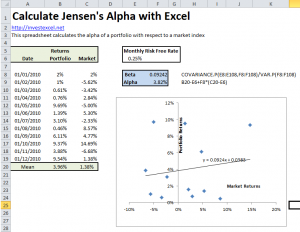According to the Capital Asset Pricing Model, Alpha is defined by this equation

alpha = rs – [rf + β (rb – rf)]

where rs is the expected portfolio return, rf is the risk-free rate, β is the portfolio beta, and rb is the market return.  Beta describes the volatility of the portfolio with respect to that of the wider market, and is calculated with this equation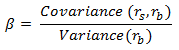The market return is usually described by the expected return of an index fund, like the FTSE or S&P500.

## Calculate Alpha with Excel

Thse steps describe how you can calculate Alpha with Excel (there’s a link to download the tutorial spreadsheet at the bottom).  The screegrabs describe the formulae used in the spreadsheet.

Step 1: Put the returns of your portfolio and the benchmark index into Excel, and calculate the average returns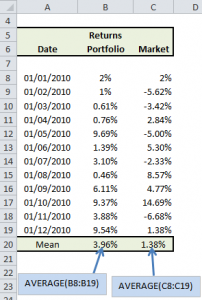Step 2. Define your risk free rate. If the returns specified in Step 1 are monthly returns, then your risk free rate has to be on a monthly basis.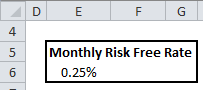Step 3. Calculate the portfolio Beta, and then the Alpha.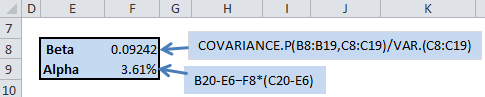### 20 thoughts on “Calculate Jensen’s Alpha with Excel”

1. The F9 cell Alpha formula is wrong : it should be “B20-E6-F8*(C20-E6)” rather than “B20-E6+F8*(C20-E6)”

• I’ve made the appropriate corrections.
Thank you for your eagle-eyed correction!

• Also, you should correct the CAPM formula for alpha: instead of “alpha = rs – rf + β (rb – rf)”, please write “alpha = rs – [rf + β (rb – rf)]”. 🙂

2. Isn’t this a monthly alpha calculation? How would you annualize?

• You annualize a monthly Jensen’s Alpha with this calculation:

(1+alpha)^12 – 1

• I calculated the monthly Alpha based on a mean monthly standard deviations, monthly risk free interest rate and beta calculated form the same data. The values work out fine.

When the monthly Alpha, the mean monthly standard deviations, and the monthly risk free interest rate are annualzed with the formula above, why does to the annualized Alpha value not Tie out using the same beta??

Thanks!

3. Your calculation is based on the assumption that the risk free rate was the same for every period which is unlikely to be the case. How would you modify the calculation to take into account varying risk free rates over the time period covered?

4. Given the monthly and yearly calculation does this then mean a daily alpha would be(1+alpha)^250-1?

5. If I have data for 9 years worth of back testing, how do I calculate the average annual alpha? Here are my inputs:
Beta: 0.88
Portfolio Return: 925%
Risk Free Return: 16.76%
Market Return:16.13%

Is calculating alpha over such a long period accepted?

6. Is it possible to find Alpha through a regression, if so how? I need to find if alpha is significant, t-stat will do.
thanks

7. Hi,

I want to use this calculator to figure out the Beta and Alpha for a portfolio on an annual basis for 2014.

What should I use for the risk free rate? 10 year treasury? Should it be the rate as of 1/1/14? Please advise.

Also if I want to annualize the Beta do I use the same formula: (1+alpha)^12 – 1

Thanks!!

8. On risk free rate, here is what you should consider, if you have access to the data: Look at a weighted average of S/T UST yields, like the weighted average yield of a 1 or 2 year bond ladder. When you talk about risk free return, short maturity treasuries are really the only securities you can talk about. The 10 YR UST does not represent credit risk, but being long the 10 YR would have hurt your account NAV during part of last year significantly.

9. Also,

Instead of running Covariance / Variance, you can run the slope function in excel which will save you steps.

10. Hi,

Suppose that I have 10 yrs of data with monthly returns, in this case which risk free rate should I use because it changes each month? Should I simply do an average?

Thank you

11. Hi,

I have monthly risk free rates of the past years. I see, in your calculation I need one value. Do I just take the mean of those risk free rates?

Further, how can I get the t-statistc to determine whether this solution is relevant?

Thank you